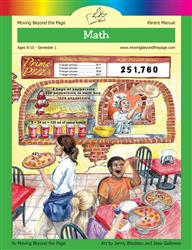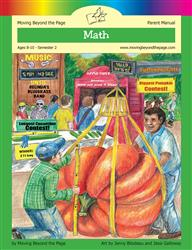# Common Core Alignment

CCSS.Math.Content.4.OA.3 - Solve multistep word problems posed with whole numbers and having whole-number answers using the four operations, including problems in which remainders must be interpreted. Represent these problems using equations with a letter standing for the unknown quantity. Assess the reasonableness of answers using mental computation and estimation strategies including rounding.

## 5: MathUnit 2: The Four Operations
Lesson 13: Problem Solving
Lesson 2: Subtraction Practice and Problem Solving
Lesson 5: Playing With the Four OperationsUnit 6: Multi-Digit Division
Lesson 3: Getting Started With Long Division
Lesson 5: Division Problem Solving
Lesson 6: Unit Test